Скачать презентацию Computation Potentials and Limitations Edward Tsang Computation

55244a5cdb34b9b9724c87f70fff4a44.ppt

• Количество слайдов: 34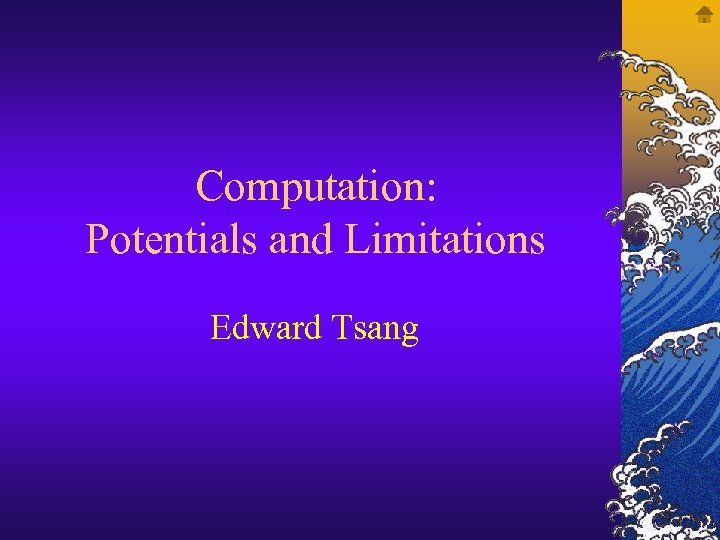Computation: Potentials and Limitations Edward Tsang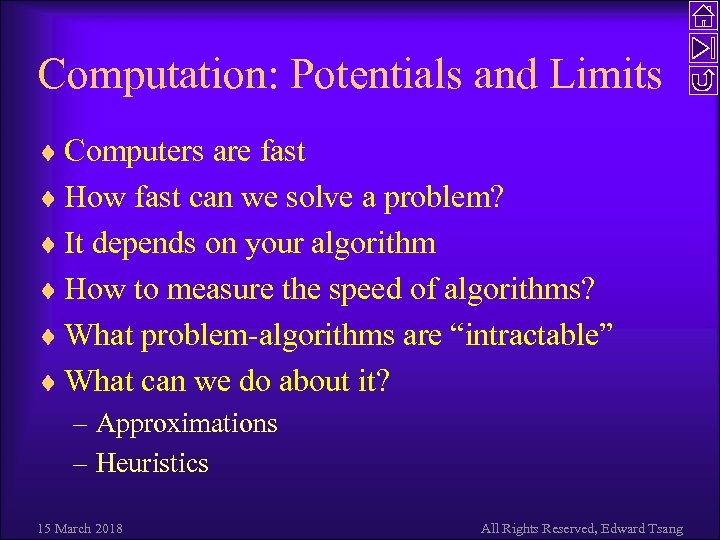Computation: Potentials and Limits ¨ Computers are fast ¨ How fast can we solve a problem? ¨ It depends on your algorithm ¨ How to measure the speed of algorithms? ¨ What problem-algorithms are “intractable” ¨ What can we do about it? – Approximations – Heuristics 15 March 2018 All Rights Reserved, Edward Tsang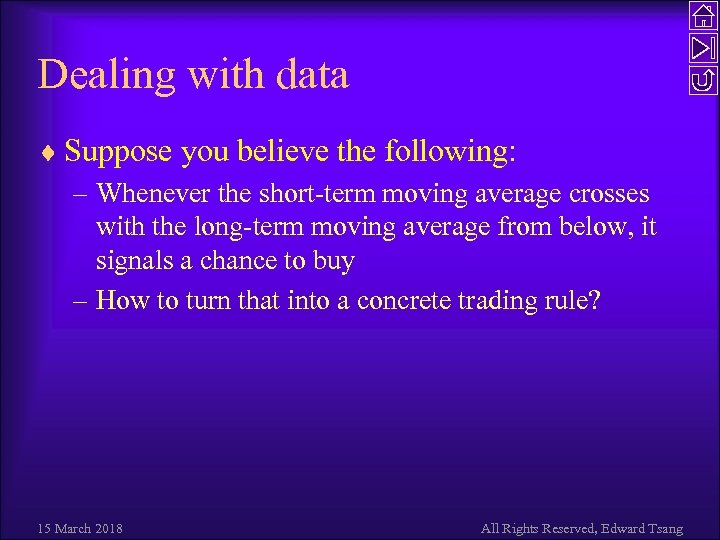Dealing with data ¨ Suppose you believe the following: – Whenever the short-term moving average crosses with the long-term moving average from below, it signals a chance to buy – How to turn that into a concrete trading rule? 15 March 2018 All Rights Reserved, Edward Tsang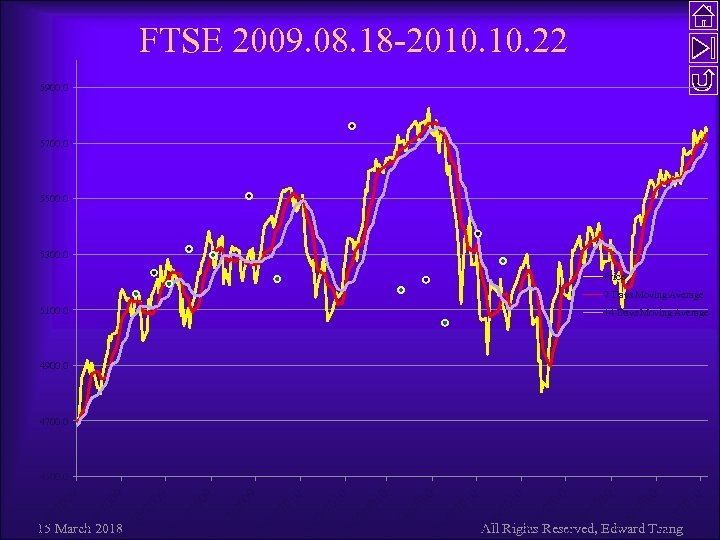FTSE 2009. 08. 18 -2010. 22 5900. 0 5700. 0 5500. 0 5300. 0 Price 7 Days Moving Average 5100. 0 14 Days Moving Average 4900. 0 4700. 0 20 10 0/ 20 10 9/ 20 10 8/ 20 10 7/ 6/ 20 10 /1 18 /0 All Rights Reserved, Edward Tsang 18 5/ 18 /0 20 10 4/ 18 /0 20 10 3/ 18 /0 20 10 2/ 18 /0 20 10 1/ 18 /0 20 09 2/ 18 /1 20 09 1/ /1 18 0/ /1 18 /0 18 18 /0 15 March 2018 20 09 9/ 8/ 20 09 4500. 0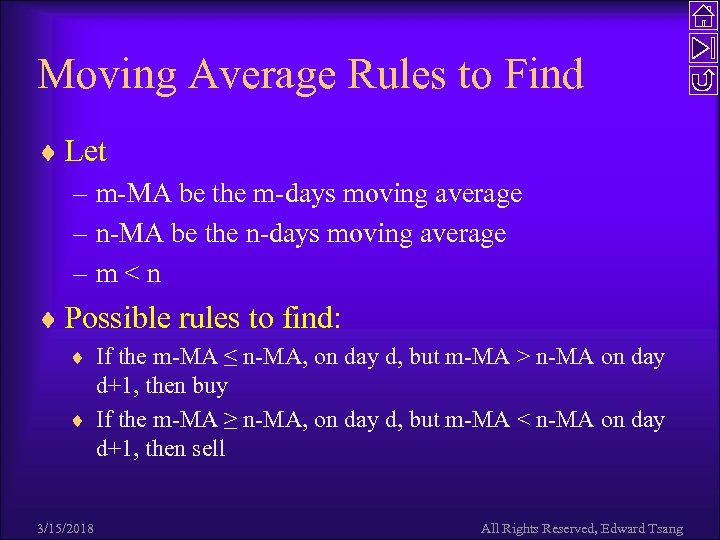Moving Average Rules to Find ¨ Let – m-MA be the m-days moving average – n-MA be the n-days moving average –m n-MA on day d+1, then buy ¨ If the m-MA ≥ n-MA, on day d, but m-MA < n-MA on day d+1, then sell 3/15/2018 All Rights Reserved, Edward Tsang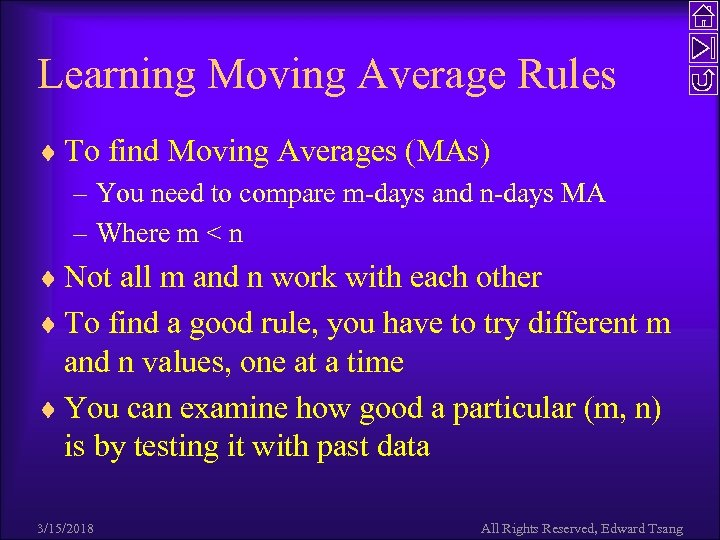Learning Moving Average Rules ¨ To find Moving Averages (MAs) – You need to compare m-days and n-days MA – Where m < n ¨ Not all m and n work with each other ¨ To find a good rule, you have to try different m and n values, one at a time ¨ You can examine how good a particular (m, n) is by testing it with past data 3/15/2018 All Rights Reserved, Edward Tsang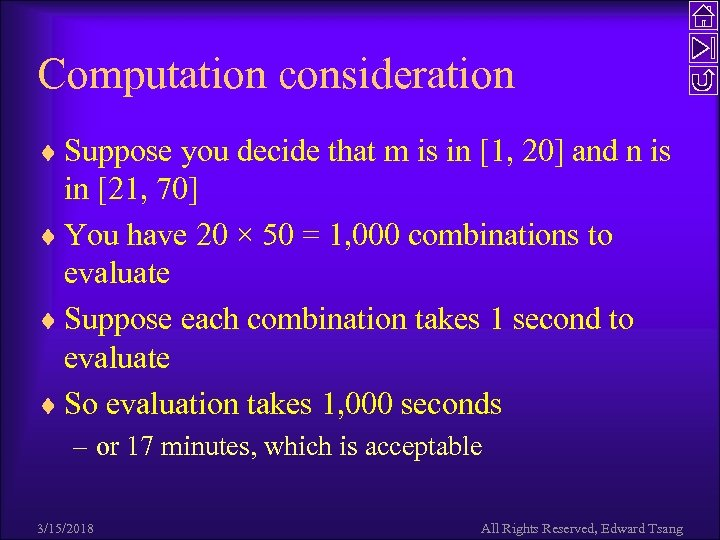Computation consideration ¨ Suppose you decide that m is in [1, 20] and n is in [21, 70] ¨ You have 20 × 50 = 1, 000 combinations to evaluate ¨ Suppose each combination takes 1 second to evaluate ¨ So evaluation takes 1, 000 seconds – or 17 minutes, which is acceptable 3/15/2018 All Rights Reserved, Edward Tsang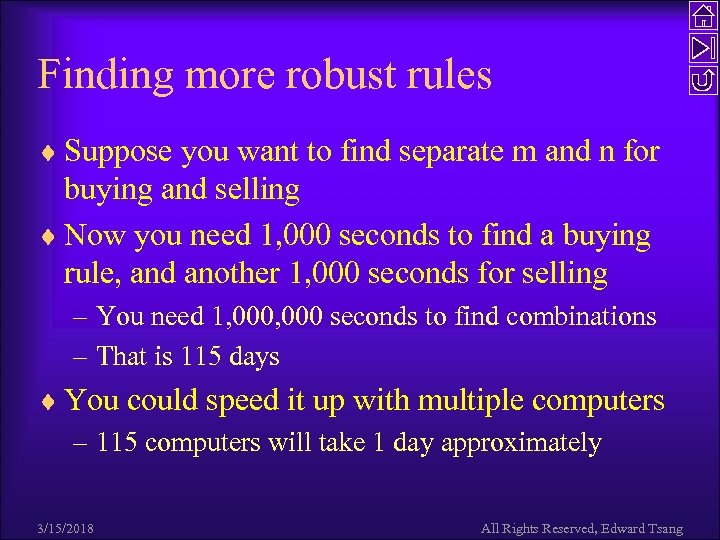Finding more robust rules ¨ Suppose you want to find separate m and n for buying and selling ¨ Now you need 1, 000 seconds to find a buying rule, and another 1, 000 seconds for selling – You need 1, 000 seconds to find combinations – That is 115 days ¨ You could speed it up with multiple computers – 115 computers will take 1 day approximately 3/15/2018 All Rights Reserved, Edward Tsang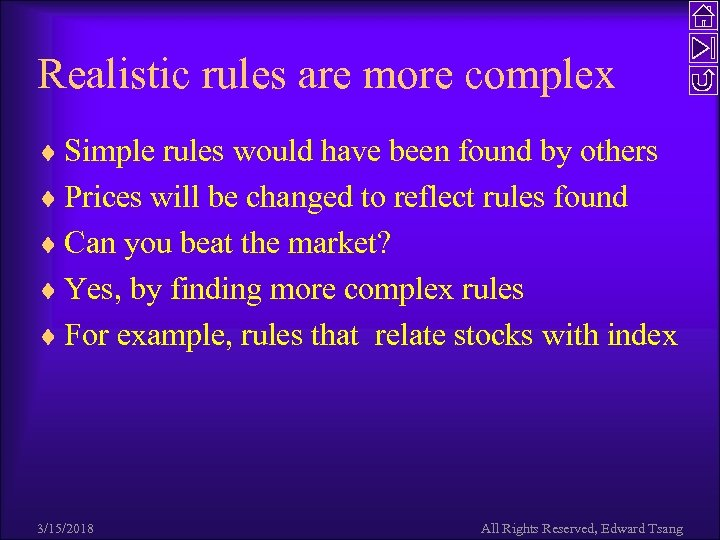Realistic rules are more complex ¨ Simple rules would have been found by others ¨ Prices will be changed to reflect rules found ¨ Can you beat the market? ¨ Yes, by finding more complex rules ¨ For example, rules that relate stocks with index 3/15/2018 All Rights Reserved, Edward Tsang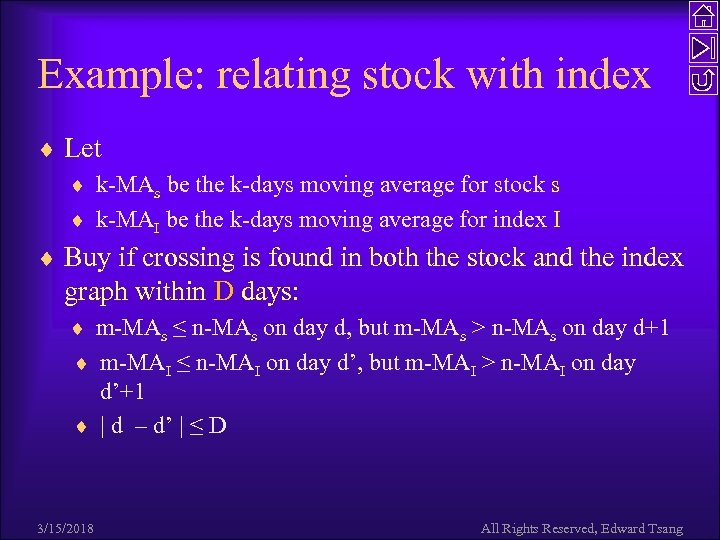Example: relating stock with index ¨ Let ¨ k-MAs be the k-days moving average for stock s ¨ k-MAI be the k-days moving average for index I ¨ Buy if crossing is found in both the stock and the index graph within D days: ¨ m-MAs ≤ n-MAs on day d, but m-MAs > n-MAs on day d+1 ¨ m-MAI ≤ n-MAI on day d’, but m-MAI > n-MAI on day d’+1 ¨ | d – d’ | ≤ D 3/15/2018 All Rights Reserved, Edward Tsang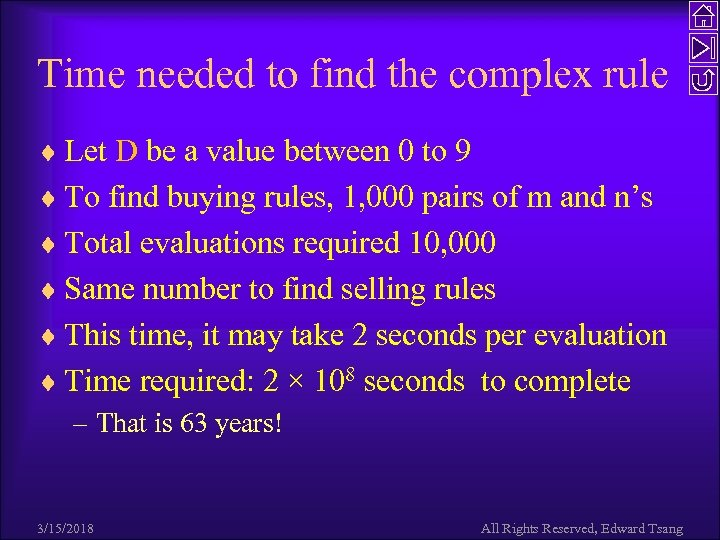Time needed to find the complex rule ¨ Let D be a value between 0 to 9 ¨ To find buying rules, 1, 000 pairs of m and n’s ¨ Total evaluations required 10, 000 ¨ Same number to find selling rules ¨ This time, it may take 2 seconds per evaluation ¨ Time required: 2 × 108 seconds to complete – That is 63 years! 3/15/2018 All Rights Reserved, Edward Tsang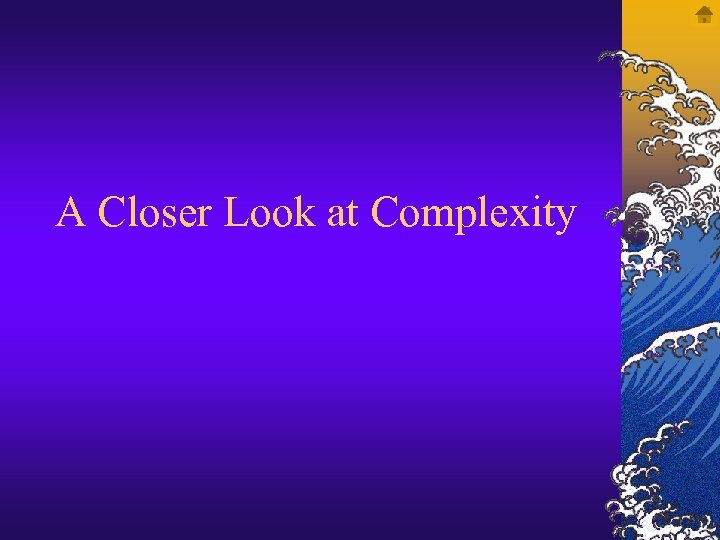A Closer Look at Complexity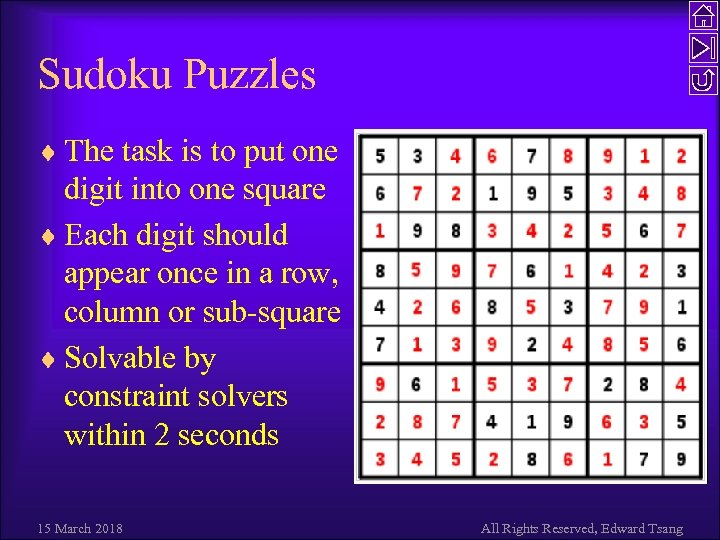Sudoku Puzzles ¨ The task is to put one digit into one square ¨ Each digit should appear once in a row, column or sub-square ¨ Solvable by constraint solvers within 2 seconds 15 March 2018 All Rights Reserved, Edward Tsang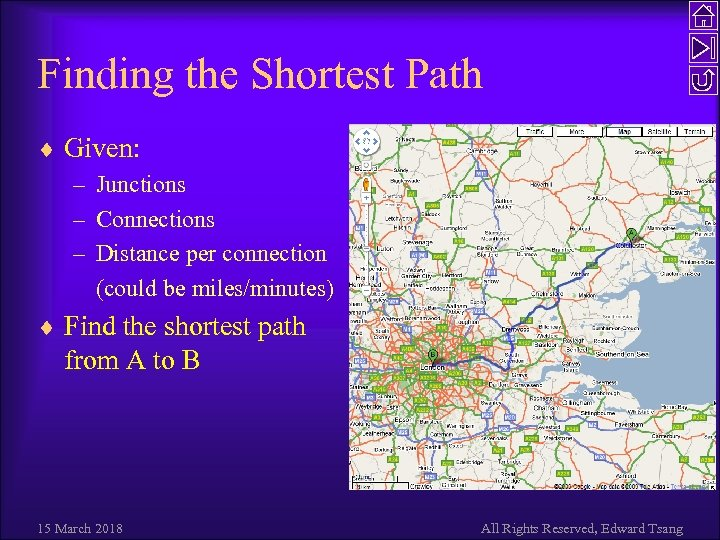Finding the Shortest Path ¨ Given: – Junctions – Connections – Distance per connection (could be miles/minutes) ¨ Find the shortest path from A to B 15 March 2018 All Rights Reserved, Edward Tsang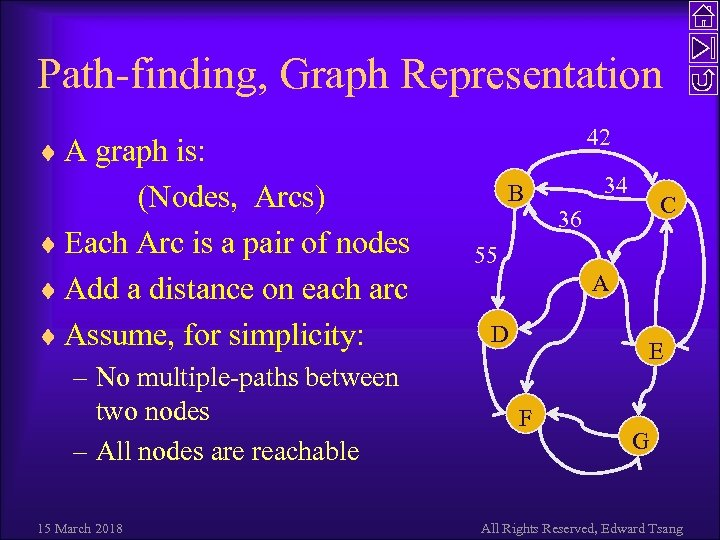Path-finding, Graph Representation 42 ¨ A graph is: (Nodes, Arcs) ¨ Each Arc is a pair of nodes ¨ Add a distance on each arc ¨ Assume, for simplicity: – No multiple-paths between two nodes – All nodes are reachable 15 March 2018 B 34 C 36 55 A D E F G All Rights Reserved, Edward Tsang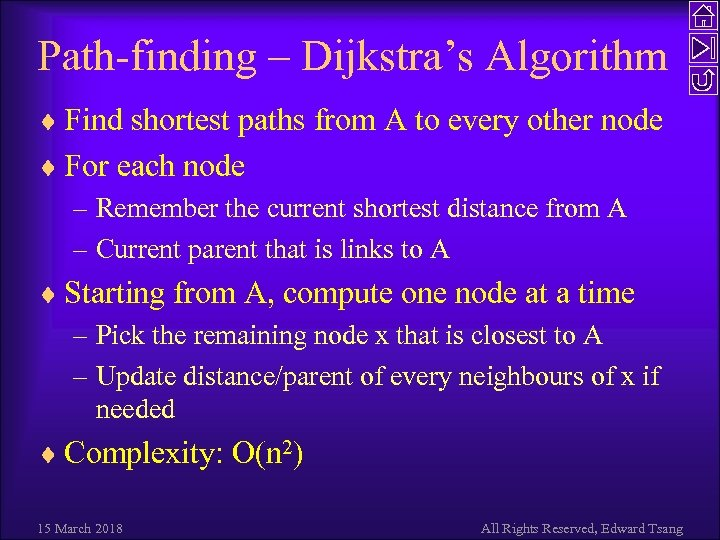Path-finding – Dijkstra’s Algorithm ¨ Find shortest paths from A to every other node ¨ For each node – Remember the current shortest distance from A – Current parent that is links to A ¨ Starting from A, compute one node at a time – Pick the remaining node x that is closest to A – Update distance/parent of every neighbours of x if needed ¨ Complexity: O(n 2) 15 March 2018 All Rights Reserved, Edward Tsang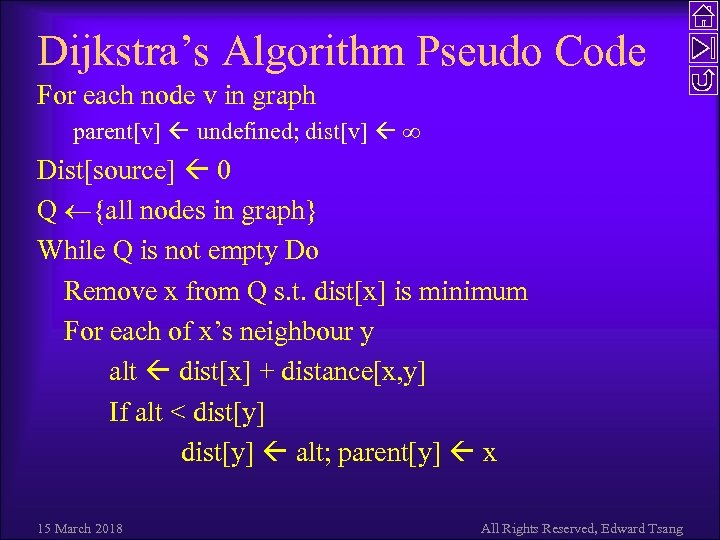Dijkstra’s Algorithm Pseudo Code For each node v in graph parent[v] undefined; dist[v] ∞ Dist[source] 0 Q {all nodes in graph} While Q is not empty Do Remove x from Q s. t. dist[x] is minimum For each of x’s neighbour y alt dist[x] + distance[x, y] If alt < dist[y] alt; parent[y] x 15 March 2018 All Rights Reserved, Edward Tsang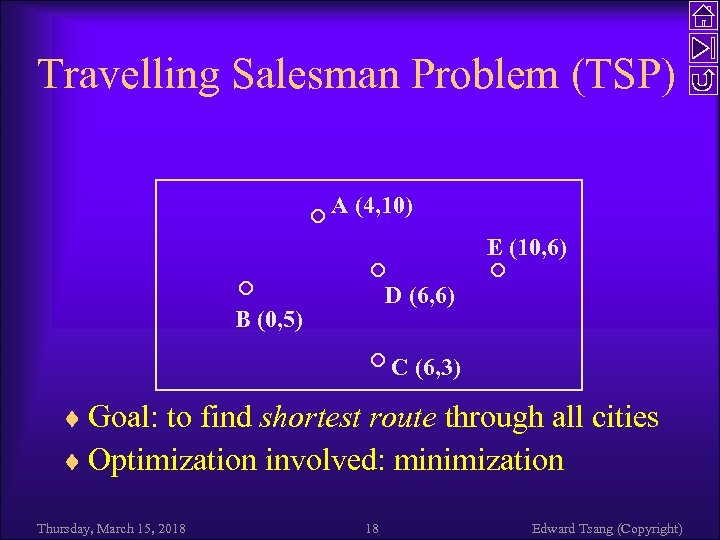Travelling Salesman Problem (TSP) A (4, 10) E (10, 6) D (6, 6) B (0, 5) C (6, 3) ¨ Goal: to find shortest route through all cities ¨ Optimization involved: minimization Thursday, March 15, 2018 18 Edward Tsang (Copyright)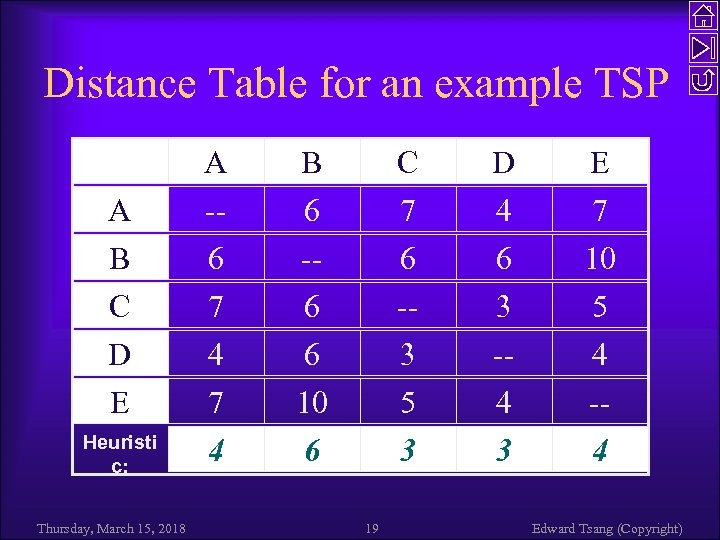Distance Table for an example TSP A B C D E Heuristi c: Thursday, March 15, 2018 A -6 7 4 B 6 -6 6 10 6 C 7 6 -3 5 3 19 D 4 6 3 -4 3 E 7 10 5 4 -4 Edward Tsang (Copyright)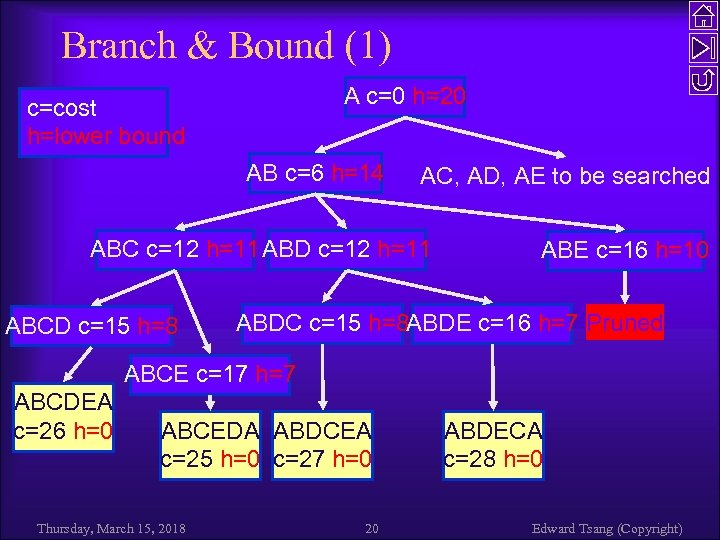Branch & Bound (1) A c=0 h=20 c=cost h=lower bound AB c=6 h=14 AC, AD, AE to be searched ABC c=12 h=11 ABD c=12 h=11 ABCD c=15 h=8 ABE c=16 h=10 ABDC c=15 h=8 ABDE c=16 h=7 Pruned ABCE c=17 h=7 ABCDEA c=26 h=0 ABCEDA ABDCEA c=25 h=0 c=27 h=0 Thursday, March 15, 2018 20 ABDECA c=28 h=0 Edward Tsang (Copyright)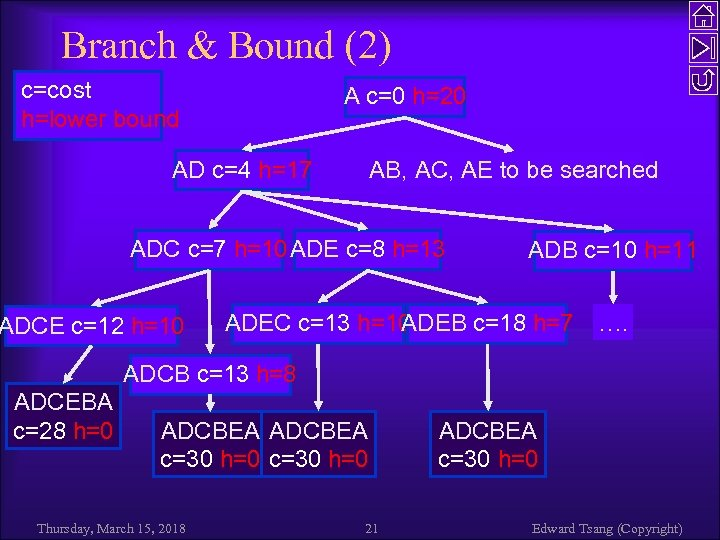Branch & Bound (2) c=cost h=lower bound A c=0 h=20 AD c=4 h=17 AB, AC, AE to be searched ADC c=7 h=10 ADE c=8 h=13 ADCE c=12 h=10 ADB c=10 h=11 ADEC c=13 h=10 ADEB c=18 h=7 …. ADCB c=13 h=8 ADCEBA c=28 h=0 ADCBEA c=30 h=0 Thursday, March 15, 2018 21 ADCBEA c=30 h=0 Edward Tsang (Copyright)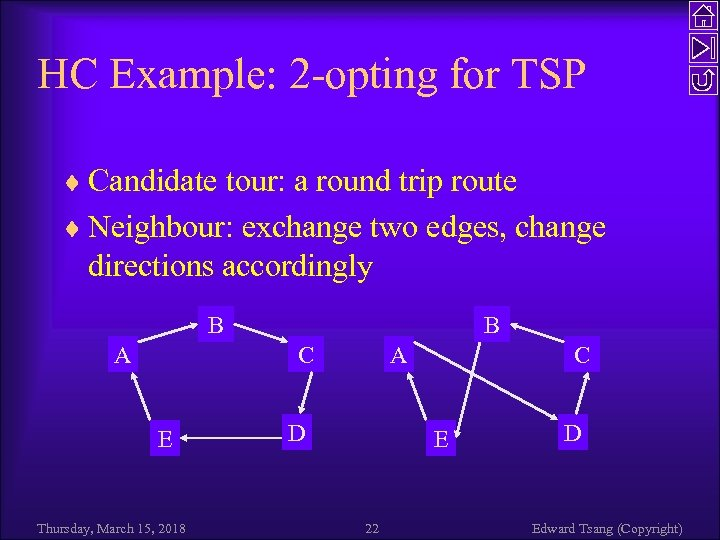HC Example: 2 -opting for TSP ¨ Candidate tour: a round trip route ¨ Neighbour: exchange two edges, change directions accordingly B A B C E Thursday, March 15, 2018 A D C E 22 D Edward Tsang (Copyright)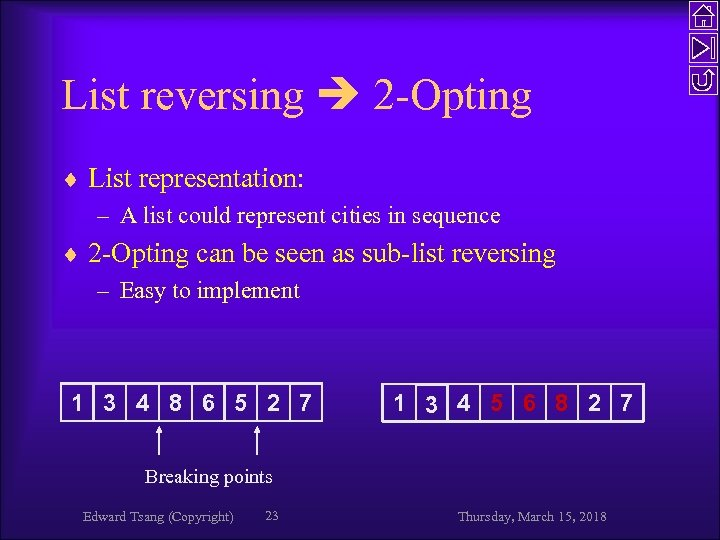List reversing 2 -Opting ¨ List representation: – A list could represent cities in sequence ¨ 2 -Opting can be seen as sub-list reversing – Easy to implement 1 3 4 8 6 5 2 7 1 3 4 5 6 8 2 7 Breaking points Edward Tsang (Copyright) 23 Thursday, March 15, 2018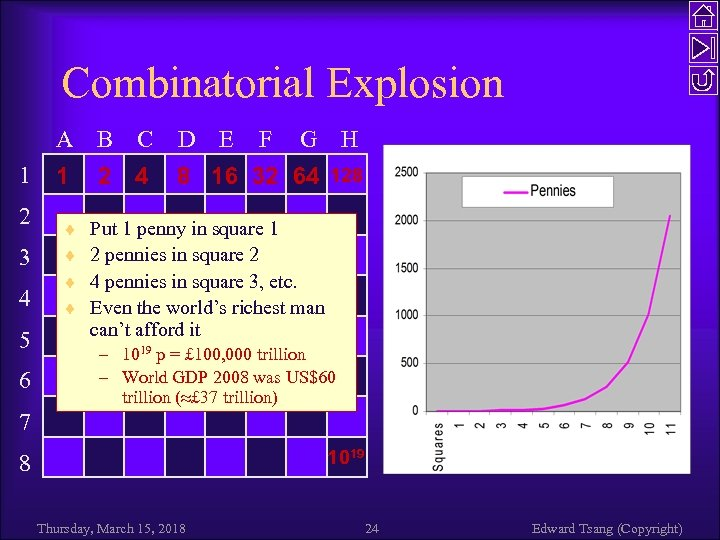Combinatorial Explosion A B 1 2 3 4 5 6 1 ¨ ¨ C 2 4 D E F G H 8 16 32 64 128 Put 1 penny in square 1 2 pennies in square 2 4 pennies in square 3, etc. Even the world’s richest man can’t afford it – 1019 p = £ 100, 000 trillion – World GDP 2008 was US\$60 trillion ( £ 37 trillion) 7 1019 8 Thursday, March 15, 2018 24 Edward Tsang (Copyright)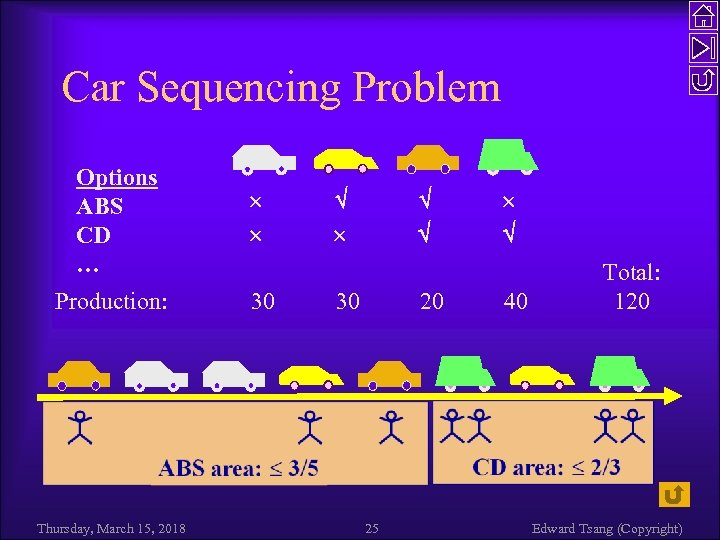Car Sequencing Problem Options ABS CD … Production: 30 Thursday, March 15, 2018 30 20 25 40 Total: 120 Edward Tsang (Copyright)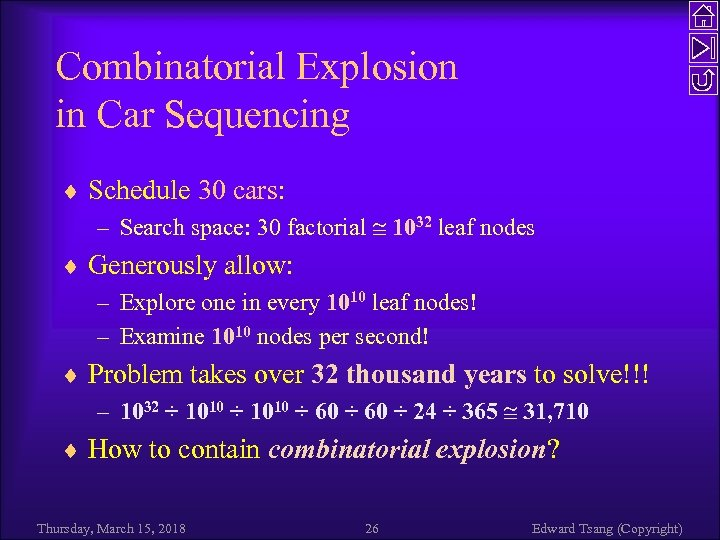Combinatorial Explosion in Car Sequencing ¨ Schedule 30 cars: – Search space: 30 factorial 1032 leaf nodes ¨ Generously allow: – Explore one in every 1010 leaf nodes! – Examine 1010 nodes per second! ¨ Problem takes over 32 thousand years to solve!!! – 1032 ÷ 1010 ÷ 60 ÷ 24 ÷ 365 31, 710 ¨ How to contain combinatorial explosion? Thursday, March 15, 2018 26 Edward Tsang (Copyright)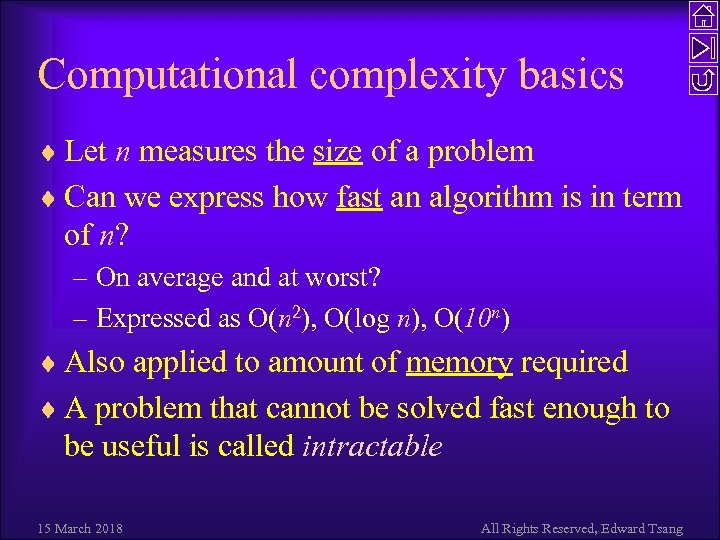Computational complexity basics ¨ Let n measures the size of a problem ¨ Can we express how fast an algorithm is in term of n? – On average and at worst? – Expressed as O(n 2), O(log n), O(10 n) ¨ Also applied to amount of memory required ¨ A problem that cannot be solved fast enough to be useful is called intractable 15 March 2018 All Rights Reserved, Edward Tsang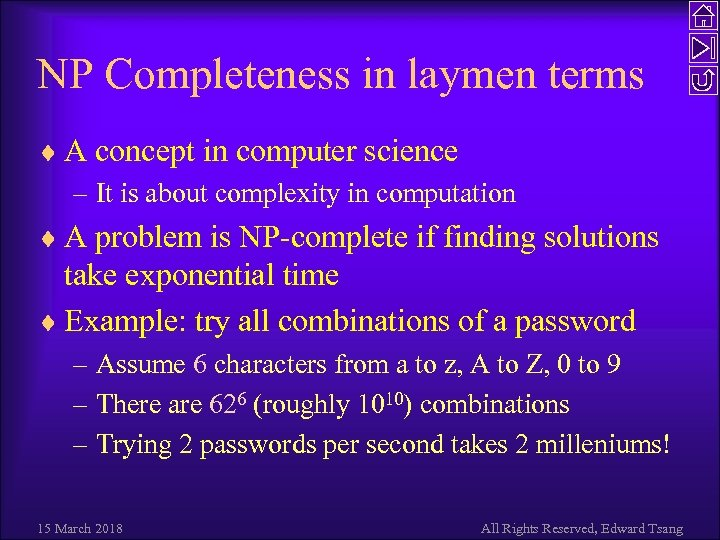NP Completeness in laymen terms ¨ A concept in computer science – It is about complexity in computation ¨ A problem is NP-complete if finding solutions take exponential time ¨ Example: try all combinations of a password – Assume 6 characters from a to z, A to Z, 0 to 9 – There are 626 (roughly 1010) combinations – Trying 2 passwords per second takes 2 milleniums! 15 March 2018 All Rights Reserved, Edward Tsang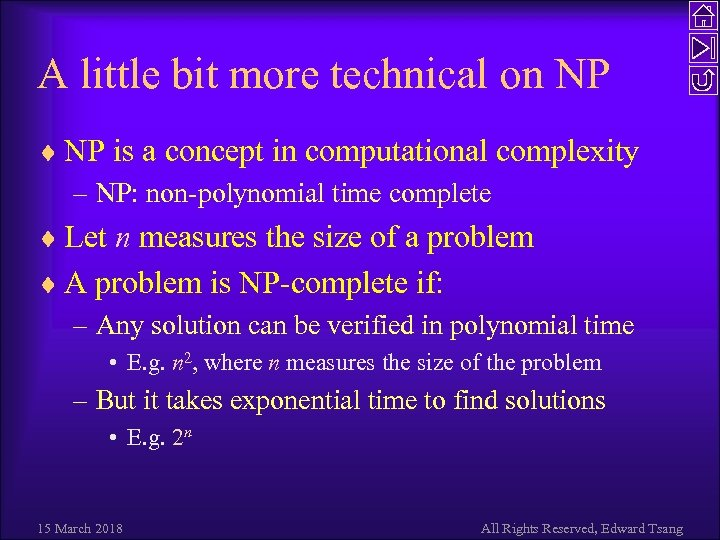A little bit more technical on NP ¨ NP is a concept in computational complexity – NP: non-polynomial time complete ¨ Let n measures the size of a problem ¨ A problem is NP-complete if: – Any solution can be verified in polynomial time • E. g. n 2, where n measures the size of the problem – But it takes exponential time to find solutions • E. g. 2 n 15 March 2018 All Rights Reserved, Edward Tsang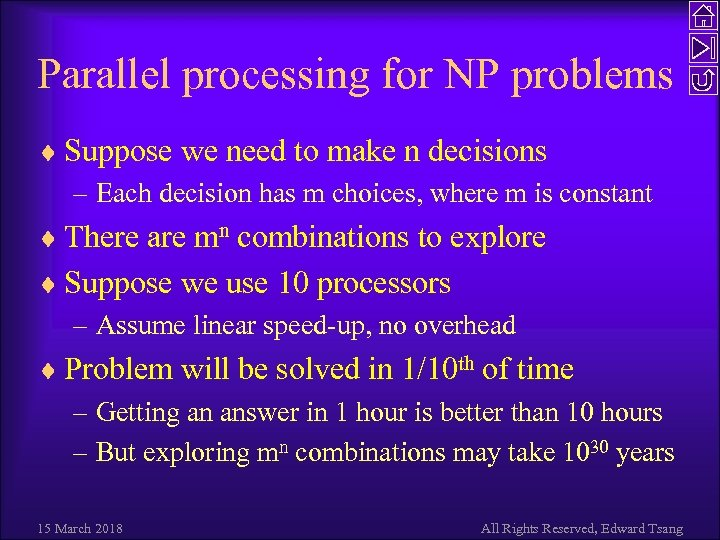Parallel processing for NP problems ¨ Suppose we need to make n decisions – Each decision has m choices, where m is constant ¨ There are mn combinations to explore ¨ Suppose we use 10 processors – Assume linear speed-up, no overhead ¨ Problem will be solved in 1/10 th of time – Getting an answer in 1 hour is better than 10 hours – But exploring mn combinations may take 1030 years 15 March 2018 All Rights Reserved, Edward Tsang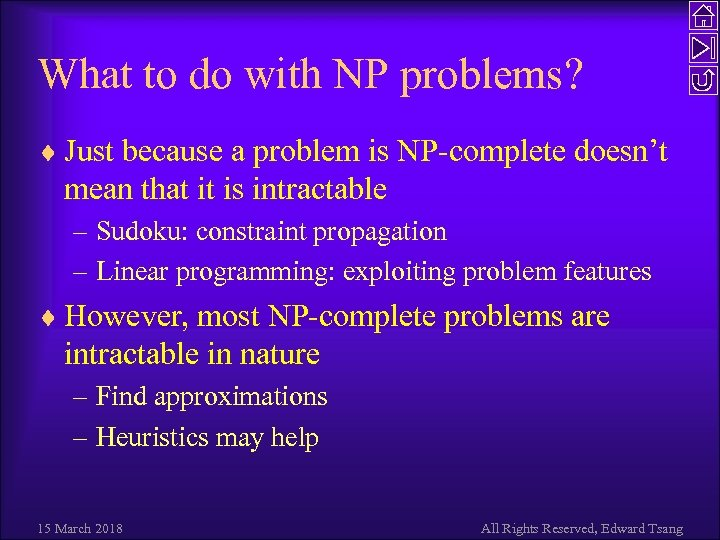What to do with NP problems? ¨ Just because a problem is NP-complete doesn’t mean that it is intractable – Sudoku: constraint propagation – Linear programming: exploiting problem features ¨ However, most NP-complete problems are intractable in nature – Find approximations – Heuristics may help 15 March 2018 All Rights Reserved, Edward Tsang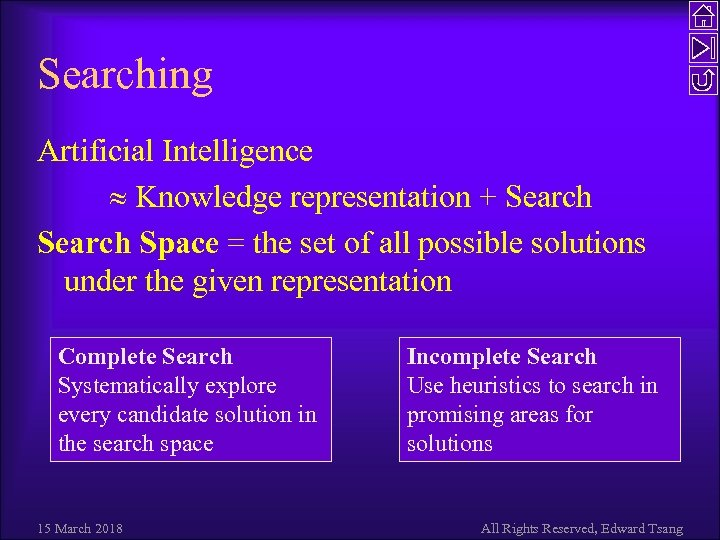Searching Artificial Intelligence Knowledge representation + Search Space = the set of all possible solutions under the given representation Complete Search Systematically explore every candidate solution in the search space 15 March 2018 Incomplete Search Use heuristics to search in promising areas for solutions All Rights Reserved, Edward Tsang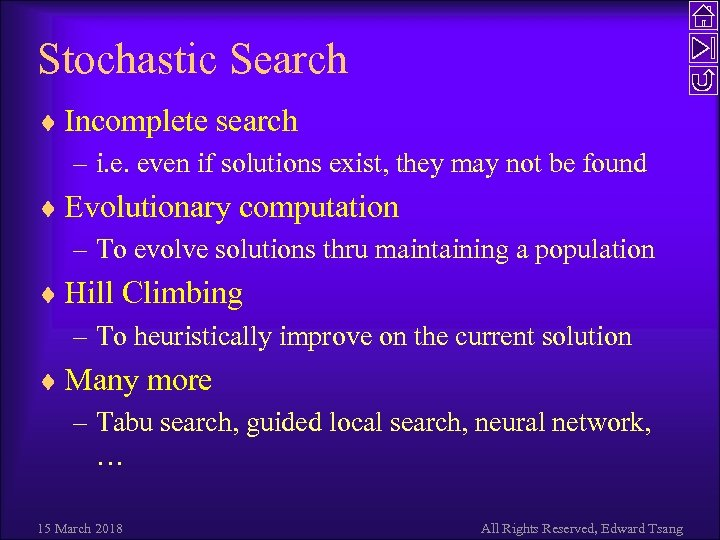Stochastic Search ¨ Incomplete search – i. e. even if solutions exist, they may not be found ¨ Evolutionary computation – To evolve solutions thru maintaining a population ¨ Hill Climbing – To heuristically improve on the current solution ¨ Many more – Tabu search, guided local search, neural network, … 15 March 2018 All Rights Reserved, Edward Tsang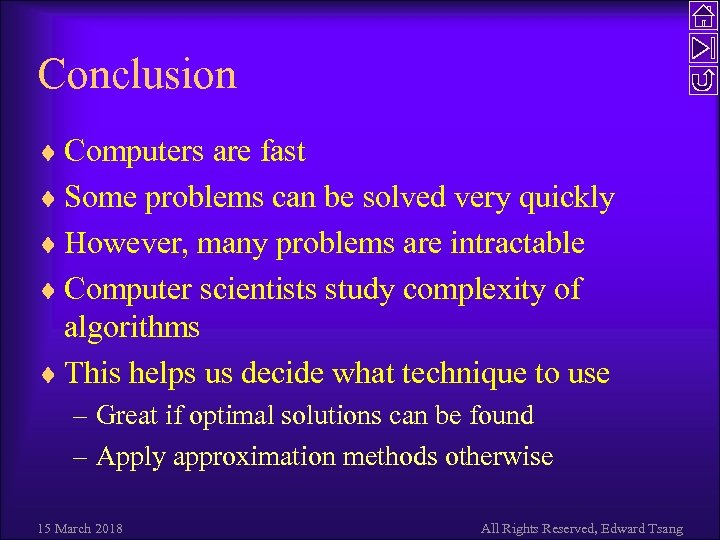Conclusion ¨ Computers are fast ¨ Some problems can be solved very quickly ¨ However, many problems are intractable ¨ Computer scientists study complexity of algorithms ¨ This helps us decide what technique to use – Great if optimal solutions can be found – Apply approximation methods otherwise 15 March 2018 All Rights Reserved, Edward Tsang